# Enum Class IntervalRelation

java.lang.Object
java.lang.Enum<IntervalRelation>
net.time4j.range.IntervalRelation
All Implemented Interfaces:
`Serializable`, `Comparable<IntervalRelation>`, `java.lang.constant.Constable`

public enum IntervalRelation extends Enum<IntervalRelation>

Represents an Allen-relation between two intervals.

Given any two intervals, there is always a unique and distinct relation between them without ambivalence. There are 13 possible relations.

 precedesmeets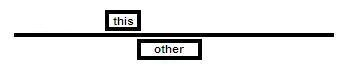overlaps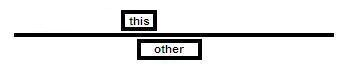finishedBy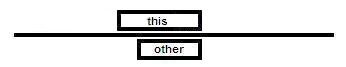encloses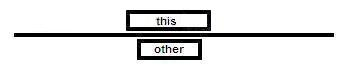starts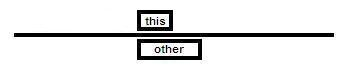equivalent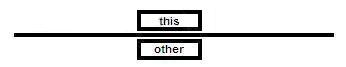startedBy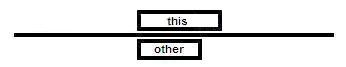enclosedBy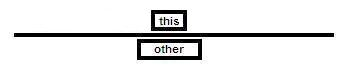finishes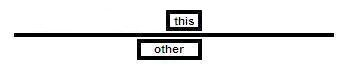overlappedBymetBy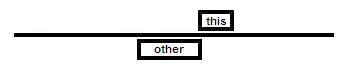precededBy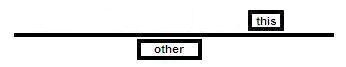Further explanations can be found at the website of Allen's interval algebra.

Since:
2.0
Author:
Meno Hochschild

## Nested classes/interfaces inherited from class java.lang.Enum

`Enum.EnumDesc<E extends Enum<E>>`
• ## Enum Constant Summary

Enum Constants
Enum Constant
Description
`ENCLOSED_BY`
`ENCLOSES`
`EQUIVALENT`
`FINISHED_BY`
`FINISHES`
`MEETS`
`MET_BY`
`OVERLAPPED_BY`
`OVERLAPS`
`PRECEDED_BY`
`PRECEDES`
`STARTED_BY`
`STARTS`
• ## Method Summary

Modifier and Type
Method
Description
`static IntervalRelation`
```between​(ClockInterval a, ClockInterval b)```
Determines the relation between given intervals.
`static IntervalRelation`
```between​(DateInterval a, DateInterval b)```
Determines the relation between given intervals.
`static IntervalRelation`
```between​(MomentInterval a, MomentInterval b)```
Determines the relation between given intervals.
`static IntervalRelation`
```between​(TimestampInterval a, TimestampInterval b)```
Determines the relation between given intervals.
`IntervalRelation`
`inverse()`
Determines the opposite relation.
`boolean`
```matches​(ClockInterval a, ClockInterval b)```
Does this relation match the relation between given intervals?
`boolean`
```matches​(DateInterval a, DateInterval b)```
Does this relation match the relation between given intervals?
`boolean`
```matches​(MomentInterval a, MomentInterval b)```
Does this relation match the relation between given intervals?
`boolean`
```matches​(TimestampInterval a, TimestampInterval b)```
Does this relation match the relation between given intervals?
`static IntervalRelation`
`valueOf​(String name)`
Returns the enum constant of this class with the specified name.
`static IntervalRelation[]`
`values()`
Returns an array containing the constants of this enum class, in the order they are declared.

### Methods inherited from class java.lang.Enum

`compareTo, describeConstable, equals, getDeclaringClass, hashCode, name, ordinal, toString, valueOf`

### Methods inherited from class java.lang.Object

`getClass, notify, notifyAll, wait, wait, wait`
• ## Enum Constant Details

• ### PRECEDES

public static final IntervalRelation PRECEDES
• ### MEETS

public static final IntervalRelation MEETS
• ### OVERLAPS

public static final IntervalRelation OVERLAPS
• ### FINISHES

public static final IntervalRelation FINISHES
• ### STARTS

public static final IntervalRelation STARTS
• ### ENCLOSES

public static final IntervalRelation ENCLOSES
• ### EQUIVALENT

public static final IntervalRelation EQUIVALENT
• ### ENCLOSED_BY

public static final IntervalRelation ENCLOSED_BY
• ### STARTED_BY

public static final IntervalRelation STARTED_BY
• ### FINISHED_BY

public static final IntervalRelation FINISHED_BY
• ### OVERLAPPED_BY

public static final IntervalRelation OVERLAPPED_BY
• ### MET_BY

public static final IntervalRelation MET_BY
• ### PRECEDED_BY

public static final IntervalRelation PRECEDED_BY
• ## Method Details

• ### values

public static  values()
Returns an array containing the constants of this enum class, in the order they are declared.
Returns:
an array containing the constants of this enum class, in the order they are declared
• ### valueOf

public static IntervalRelation valueOf(String name)
Returns the enum constant of this class with the specified name. The string must match exactly an identifier used to declare an enum constant in this class. (Extraneous whitespace characters are not permitted.)
Parameters:
`name` - the name of the enum constant to be returned.
Returns:
the enum constant with the specified name
Throws:
`IllegalArgumentException` - if this enum class has no constant with the specified name
`NullPointerException` - if the argument is null
• ### inverse

public IntervalRelation inverse()

Determines the opposite relation.

Returns:
opposite relation
Since:
2.0
• ### matches

public boolean matches

Does this relation match the relation between given intervals?

Parameters:
`a` - first interval
`b` - second interval
Returns:
`true` if both intervals have this relation to each other else `false`
Since:
2.0
• ### matches

public boolean matches

Does this relation match the relation between given intervals?

Parameters:
`a` - first interval
`b` - second interval
Returns:
`true` if both intervals have this relation to each other else `false`
Since:
2.0
• ### matches

public boolean matches

Does this relation match the relation between given intervals?

Parameters:
`a` - first interval
`b` - second interval
Returns:
`true` if both intervals have this relation to each other else `false`
Since:
2.0
• ### matches

public boolean matches

Does this relation match the relation between given intervals?

Parameters:
`a` - first interval
`b` - second interval
Returns:
`true` if both intervals have this relation to each other else `false`
Since:
2.0
• ### between

public static IntervalRelation between

Determines the relation between given intervals.

Parameters:
`a` - first interval
`b` - second interval
Returns:
relation between given intervals
Since:
2.0
• ### between

public static IntervalRelation between

Determines the relation between given intervals.

Parameters:
`a` - first interval
`b` - second interval
Returns:
relation between given intervals
Since:
2.0
• ### between

public static IntervalRelation between

Determines the relation between given intervals.

Parameters:
`a` - first interval
`b` - second interval
Returns:
relation between given intervals
Since:
2.0
• ### between

public static IntervalRelation between

Determines the relation between given intervals.

Parameters:
`a` - first interval
`b` - second interval
Returns:
relation between given intervals
Since:
2.0